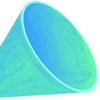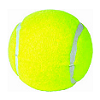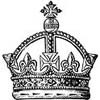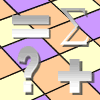# Search by Topic

Filter by: Content type:
Age range:
Challenge level:

### There are 68 results

Broad Topics > Information and Communications Technology > Spreadsheets### What's the Weather Like?

##### Age 11 to 14 Challenge Level:

With access to weather station data, what interesting questions can you investigate?### Time to Evolve 2

##### Age 16 to 18 Challenge Level:

How is the length of time between the birth of an animal and the birth of its great great ... great grandparent distributed?### The Legacy

##### Age 14 to 16 Challenge Level:

Your school has been left a million pounds in the will of an ex- pupil. What model of investment and spending would you use in order to ensure the best return on the money?### Funnel

##### Age 14 to 16 Challenge Level:

A plastic funnel is used to pour liquids through narrow apertures. What shape funnel would use the least amount of plastic to manufacture for any specific volume ?### Cola Can

##### Age 11 to 14 Challenge Level:

An aluminium can contains 330 ml of cola. If the can's diameter is 6 cm what is the can's height?### Negative Power

##### Age 14 to 16 Challenge Level:

What does this number mean ? Which order of 1, 2, 3 and 4 makes the highest value ? Which makes the lowest ?### Minus One Two Three

##### Age 14 to 16 Challenge Level:

Substitute -1, -2 or -3, into an algebraic expression and you'll get three results. Is it possible to tell in advance which of those three will be the largest ?### Dating Made Easier

##### Age 14 to 16 Challenge Level:

If a sum invested gains 10% each year how long before it has doubled its value?### Factor-multiple Chains

##### Age 7 to 11 Challenge Level:

Can you see how these factor-multiple chains work? Find the chain which contains the smallest possible numbers. How about the largest possible numbers?### Multiples Grid

##### Age 7 to 11 Challenge Level:

What do the numbers shaded in blue on this hundred square have in common? What do you notice about the pink numbers? How about the shaded numbers in the other squares?### Take Ten Sticks

##### Age 11 to 16 Challenge Level:

Take ten sticks in heaps any way you like. Make a new heap using one from each of the heaps. By repeating that process could the arrangement 7 - 1 - 1 - 1 ever turn up, except by starting with it?### Substitution Cipher

##### Age 11 to 14 Challenge Level:

Find the frequency distribution for ordinary English, and use it to help you crack the code.### Litov's Mean Value Theorem

##### Age 11 to 14 Challenge Level:

Start with two numbers and generate a sequence where the next number is the mean of the last two numbers...### Sending a Parcel

##### Age 11 to 14 Challenge Level:

What is the greatest volume you can get for a rectangular (cuboid) parcel if the maximum combined length and girth are 2 metres?### Odd Differences

##### Age 14 to 16 Challenge Level:

The diagram illustrates the formula: 1 + 3 + 5 + ... + (2n - 1) = n² Use the diagram to show that any odd number is the difference of two squares.### Training Schedule

##### Age 14 to 16 Challenge Level:

The heptathlon is an athletics competition consisting of 7 events. Can you make sense of the scoring system in order to advise a heptathlete on the best way to reach her target?### Any Win for Tennis?

##### Age 16 to 18 Challenge Level:

What are your chances of winning a game of tennis?### Succession in Randomia

##### Age 16 to 18 Challenge Level:

By tossing a coin one of three princes is chosen to be the next King of Randomia. Does each prince have an equal chance of taking the throne?### Percentages for Key Stage 4

##### Age 14 to 16 Challenge Level:

A group of interactive resources to support work on percentages Key Stage 4.### Peaches in General

##### Age 14 to 16 Challenge Level:

It's like 'Peaches Today, Peaches Tomorrow' but interestingly generalized.### Zeller's Birthday

##### Age 14 to 16 Challenge Level:

What day of the week were you born on? Do you know? Here's a way to find out.### Unusual Long Division - Square Roots Before Calculators

##### Age 14 to 16 Challenge Level:

However did we manage before calculators? Is there an efficient way to do a square root if you have to do the work yourself?### The Fire-fighter's Car Keys

##### Age 14 to 16 Challenge Level:

A fire-fighter needs to fill a bucket of water from the river and take it to a fire. What is the best point on the river bank for the fire-fighter to fill the bucket ?.### Under the Ribbon

##### Age 14 to 16 Challenge Level:

A ribbon is nailed down with a small amount of slack. What is the largest cube that can pass under the ribbon ?### Pythagoras’ Comma

##### Age 14 to 16 Challenge Level:

Using an understanding that 1:2 and 2:3 were good ratios, start with a length and keep reducing it to 2/3 of itself. Each time that took the length under 1/2 they doubled it to get back within range.### Excel Interactive Resource: Haitch

##### Age 11 to 16 Challenge Level:

An Excel spreadsheet with an investigation.### Getting to Know Excel

##### Age 11 to 16 Challenge Level:

A number of useful techniques that can extend your use of Excel.### Excel Investigation: Sums of Consecutive Numbers

##### Age 11 to 16 Challenge Level:### Excel Investigation: Pythagorean Triples

##### Age 11 to 16 Challenge Level:

Use Excel to find sets of three numbers so that the sum of the squares of the first two is equal to the square of the third.### Excel Technique: Composite Bar Charts

##### Age 11 to 16 Challenge Level:

Learn how to use composite bar charts in Excel.### Excel Investigation: Multiples of Three

##### Age 11 to 16 Challenge Level:

Use Excel to investigate digit sums of multiples of three. Can you explain your findings?### Excel Investigation: Beads

##### Age 11 to 16 Challenge Level:

Use Excel to investigate remainders and divisors. Was your result predictable?### Excel Technique: LCM & HCF (or GCD)

##### Age 11 to 16 Challenge Level:

Learn how to use the Excel functions LCM and GCD.### Excel Interactive Resource: Fraction Multiplication

##### Age 11 to 16 Challenge Level:

Use Excel to explore multiplication of fractions.### Excel Interactive Resource: Fraction Addition & Fraction Subtraction

##### Age 11 to 16 Challenge Level:

Use Excel to practise adding and subtracting fractions.### Excel Investigation: Fraction Combinations

##### Age 11 to 16 Challenge Level:

Choose four numbers and make two fractions. Use an Excel spreadsheet to investigate their properties. Can you generalise?### Excel Investigation: Difference Tuples

##### Age 11 to 16 Challenge Level:

Use an Excel spreadsheet to investigate differences between four numbers. Which set of start numbers give the longest run before becoming 0 0 0 0?### Excel Technique: Logic Tests

##### Age 11 to 16 Challenge Level:

Learn how to use logic tests to create interactive resources using Excel.### Excel Interactive Resource: the up and Down Game

##### Age 11 to 16 Challenge Level:

Use an interactive Excel spreadsheet to explore number in this exciting game!### Excel Interactive Resource: Multiples Chain

##### Age 11 to 16 Challenge Level:

Use an interactive Excel spreadsheet to investigate factors and multiples.### Excel Investigation: Target Decimal

##### Age 11 to 16 Challenge Level:

Use an Excel spreadsheet to approximate a decimal using trial and improvement.### Excel Investigation: Planks

##### Age 11 to 16 Challenge Level:

I have an unlimited supply of planks, of lengths 7 and 9 units. Putting planks end to end, what total lengths can be achieved? Use Excel to investigate.### Excel Technique: LOOKUP Functions

##### Age 11 to 16 Challenge Level:

Learn how to use lookup functions to create exciting interactive Excel spreadsheets.### Excel Interactive Resource: Equivalent Fraction Bars

##### Age 11 to 16 Challenge Level:

A simple file for the Interactive whiteboard or PC screen, demonstrating equivalent fractions.### Excel Interactive Resource: Long Multiplication

##### Age 11 to 16 Challenge Level:

Use an Excel spreadsheet to explore long multiplication.### Excel Investigation: Happy Numbers

##### Age 11 to 16 Challenge Level:

Take any whole number between 1 and 999, add the squares of the digits to get a new number. Use a spreadsheet to investigate this sequence.### Excel Technique: Triangular Arrays by Turning Off Zeros

##### Age 11 to 16 Challenge Level:

Learn how to use Excel to create triangular arrays.### Excel Interactive Resource: Number Grid Functions

##### Age 11 to 16 Challenge Level:

Use Excel to investigate the effect of translations around a number grid.### Excel Investigation: Power Crazy

##### Age 11 to 16 Challenge Level:

When is 7^n + 3^n a multiple of 10? Use Excel to investigate, and try to explain what you find out.### Excel Investigation: Pascal Multiples

##### Age 11 to 16 Challenge Level:

This spreadsheet highlights multiples of numbers up to 20 in Pascal's triangle. What patterns can you see?